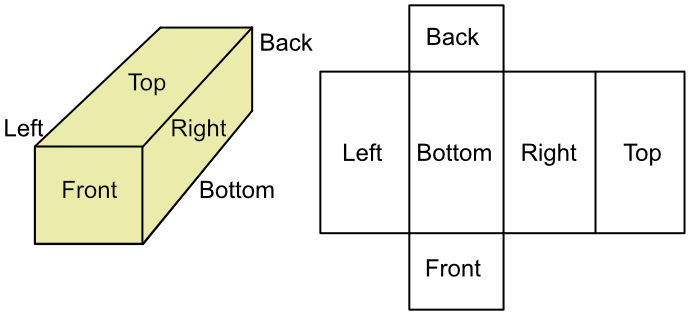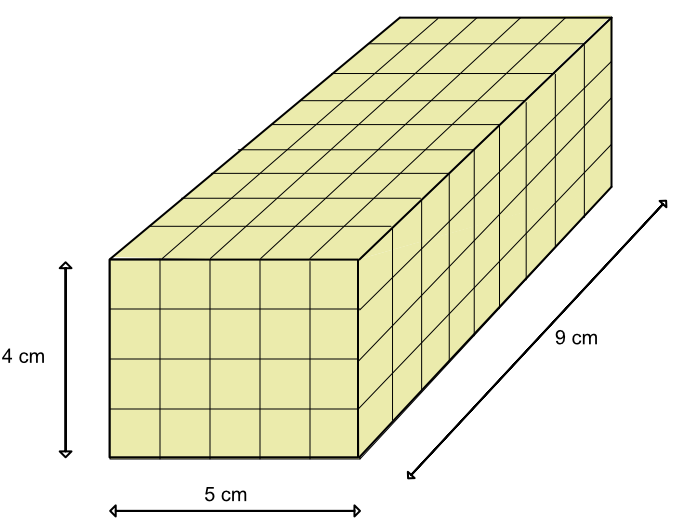Surface Area of a Cuboid

## Surface Area of a Cuboid

Each face on a cuboid consists of an area. The Surface Area of a cuboid is the total of all the areas on each face added together. The same area is obtained when a net of the shape is created.A cuboid has 6 surfaces: a front and back, a left and right, and a top and bottom. Each pair of surfaces has the same area.

Calculate the area for each surface, then add the areas together for the total surface area. It is important to include all the surfaces, including any hidden surfaces.

Note that the surface area, like all areas, is a square unit e.g. cm2, m2.

## Example 1

What is the surface area of a cuboid that is 5cm wide, 4cm tall and 9 cm long?Front: 4 x 5 = 20 Back: 4 x 5 = 20 Left: 4 x 9 = 36 Right: 4 x 9 = 36 Top: 5 x 9 = 45 Bottom: 5 x 9 = 45 TOTAL = 202

 Surface Area = front + back + left + right + top + bottom 1000 = 10 x 10 + 10 x 10 + 10xx + 10 x x + 10 x x + 10 x x = 200 + 40x 800 = 40x 20 = x